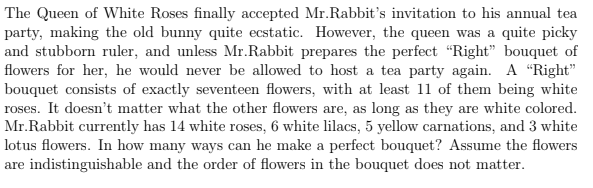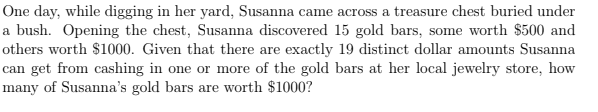# Q's from math competition

• Hey all, I am trying out some question samples for a competition I am interested in participating in, and there are two questions I don't know how to solve. Could ya' all help me out a bit, maybe first off by giving me some hint so I could try to work on it again??! Thanks a lot! oh, here is the question:

I know this involves combinatorics, and I really want to use multiplication to times everything together, but I don't know how to do it and I don't even know if it is the correct way to answer this question!for this question, I think the reason I don't know how to solve it is that I don't know how to use the third information, which is she uses exactly 19 distinct dollar amounts, what does this help me to reach the answer?• Great questions!

For #1, since all the flowers in the bouquet have to be white, they have to be either white roses, white lilacs, or white lotus flowers, and there must be 17 total flowers. Furthermore, the #white roses must be at most 14, the #white lilacs must be at most 6, and the #white lotus flowers must be at most 3. We will look at several different cases:

11 white roses: # white lilacs + #white lotus flowers = 6, so (#white lilacs, #white lotus flowers) = any of (6, 0), (5, 1), (4, 2), (3, 3) ==> a total of 4 options.

12 white roses: # white lilacs + #white lotus flowers = 5, so (#white lilacs, #white lotus flowers) = any of (5, 0), (4, 1), (3, 2), (2, 3) ==> a total of 4 options.

13 white roses: # white lilacs + #white lotus flowers = 4, so (#white lilacs, #white lotus flowers) = any of (4, 0), (3, 1), (2, 2), (1, 3) ==> a total of 4 options.

14 white roses: # white lilacs + #white lotus flowers = 3, so (#white lilacs, #white lotus flowers) = any of (3, 0), (2, 1), (1, 2), (0, 3) ==> a total of 4 options.

Therefore, there are a total of 4+4+4+4 = 16 perfect bouquets.

Note: It seems tempting to multiply everything together, since you have 4 choices for the #white roses (11, 12, 13, 14) and 7 choices for the #white lilacs (0, 1, 2, 3, 4, 5, 6) and 4 choices for the #white lotus flowers (0, 1, 2, 3) but then there's the constraint of exactly 17 flowers total, which makes everything messy.I wish there was a non-bash solution, and I'd be very interested if there is one!For #2, there are some key observations:

1. All the dollar amounts Susanna can get from cashing in one or more of her gold bars are multiples of 500. (This is because the value of each gold bar is a multiple of 500.)

2. If m is the total value of all of Susanna's gold bars, by cashing in gold bars, Susanna can get any multiple of 500 from 500 up to m.
This is a very tricky observation! There's actually a very strategic way to get every multiple. Let's take 5 500's and 4 1000's for example. Then we can get all the multiples of 1000 up to 4*1000 by just using 1000's, and we can also get 500, 1000+500, 2000+500, 3000+500, 4000+500 from by just adding 500 to multiples of 1000. Furthermore, we can get numbers greater than 4000+500 by adding 500's like this (4000+1000, 4000+1500, 4000+2000, 4000+2500), until we reach the ultimate maximum, 4000+2500. And so we can reach all the possible multiples of 500!

So if x is the number of \$500 gold bars, and 15 - x is the number of \$1000 gold bars, then m = 500(x) + 1000(15 - x) = 500(x + 30 - 2x) = 500(30 - x).
If there are 19 multiples of 500 between 500(1) and 500(30 - x) inclusive, then 30 - x = 19, so x = 11, and 15 - x = 4, which is the number of gold bars worth \$1000.

I hope this made sense! Let me know if anything is unclear, or if my answers are wrong.• @victorioussheep What contest is this? Just curious• @v4913

I can't thank you enough for all the work and time you have put in to answer my question! I think I have now understood the first question, but I will try to solve it on my own tomorrow without looking at your explanation and see if I truly understand the logic behind it!

For the second question, I can understand how you come up with the final equation, which is m = 500x + 1000(15-x), but I still can't understand how the 19 is being used in the question, and how can you know by looking at the question what the "tricky observation" is? Is there any keyword that I missed out on while I was reading the question?

• That's great!Oh yeah, sorry, that was a bit confusing- but basically the tricky observation is not unique to this problem; it's a pretty general fact that if you have objects worth n and 2n, you can use them to make any multiple of n up to the total of all of your objects. And then the 19 is just something given in the problem which you can use the tricky observation on to solve the problem. The 19 is not actually related to finding out the tricky observation.As for how the 19 is being used in the question, because of the tricky observation, you know that the total value of all the gold bars must be 500*19, so that Susanna will be able to obtain 19 different values:
500 * 1
500 * 2
...
500 * 18
500 * 19
And then you can use that to figure out how many of each type of gold bar there are. For example, if the problem said 17 instead of 19, there would be 17 values that Susanna could obtain, and they would have to be these:
500 * 1
500 * 2
...
500 * 16
500 * 17
Then using systems of equations, you would get that there are 13 \$500 gold bars and 2 \$1000 gold bars, because 13 * 500 + 2 * 1000 = 17 * 500.

• @v4913

Thanks for the reply, I think I have understood how to solve the two problems, with special thanks to you!

• No problem! Thanks for asking them!